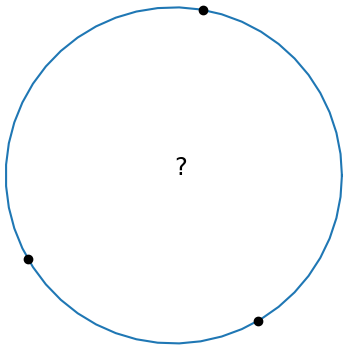# Equation of a circle through three pointsA few months ago I wrote about several equations that come up in geometric calculations that all have the form of a determinant equal to 0, where one column of the determinant is all 1s.

The equation of a circle through three points (x1, y1), (x2, y2), and (x3, y3) has this form:This is elegant equation, but it’s not in a form that’s ready to program. This post will start with the equation above and conclude with code.

Let Mij be the determinant of the minor of the (i, j) element of the matrix above. That is, Mij is the determinant of the 3 × 3 matrix obtained by deleting the ith row and jth column. Then we can expand the determinant above by minors of the last column to getwhich we can rewrite asA circle with center (x0, y0) and radius r0 has equationBy equating the coefficients x and y in the two previous equations we haveNext we expand the determinant minors in more explicit form.Having gone to the effort of finding a nice expression for M11 we can reuse it to find M12 by the substitutionas follows:And we can find M13 from M12 by reversing the role of the xs and ys.Now we have concrete expressions for M11, M12, and M13, and these give us concrete expressions for x0 and y0.

To find the radius of the circle we simply find the distance from the center (x0, y0) to any of the points on the circle, say (x1, y1).

The following Python code incorporates the derivation above.

    def circle_thru_pts(x1, y1, x2, y2, x3, y3):
s1 = x1**2 + y1**2
s2 = x2**2 + y2**2
s3 = x3**2 + y3**2
M11 = x1*y2 + x2*y3 + x3*y1 - (x2*y1 + x3*y2 + x1*y3)
M12 = s1*y2 + s2*y3 + s3*y1 - (s2*y1 + s3*y2 + s1*y3)
M13 = s1*x2 + s2*x3 + s3*x1 - (s2*x1 + s3*x2 + s1*x3)
x0 =  0.5*M12/M11
y0 = -0.5*M13/M11
r0 = ((x1 - x0)**2 + (y1 - y0)**2)**0.5
return (x0, y0, r0)


The code doesn’t use any loops or arrays, so it ought to be easy to port to any programming language.

For some inputs the value of M11 can be (nearly) zero, in which case the three points (nearly) lie on a line. In practice M11 can be nonzero and yet zero for practical purposes and so that’s something to watch out for when using the code.

The determinant at the top of the post is zero when the points are colinear. There’s a way to make rigorous the notion that in this case the points line on a circle centered at infinity.

## 2 thoughts on “Equation of a circle through three points”

1. Richard Pennington

It may be easier to take two perpendicular bisectors to find the centre, then draw the circle from there.

Meanwhile, I had an interesting case: it is possible to draw an ellipse of any aspect ratio and orientation through any set of three points (proof: affine transformation to make it a circle, then draw the circle and perform the inverse affine transformation). Testing this out with three “random” points and keeping the aspect ratio constant while varying the orientation, I found that the ellipses all passed through *four* common points (my original three plus an extra). A sample of one, but it may well be worth investigation.

2. Richard Pennington

Another thought: the condition for NOT being able to draw an ellipse through four points appears to be that one of the points lies inside the triangle defined by the other three points. If three of the points are collinear, the resulting conic is degenerate.